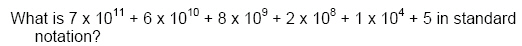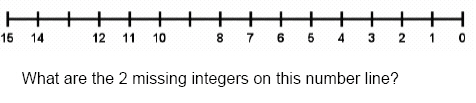Multiple Choice
Identify the choice that best completes the statement or answers the question.

1.

Number Sense and Numeration – Percents – RIT 201 – 210
What is 60% of 20?
 a. 120 d. 60 b. 12 e. 10 c. 80

2.

1/8 expressed as a percent is:
 a. 125% d. 1.25% b. 12.5% e. 12% c. 0.125%

3.

Number Sense and Numeration – Place Value – RIT 201 – 210
2,478,015,963
Which digit is in the ten-millions place?
 a. 4 d. 6 b. 7 e. 8 c. 1

4.

Which decimal represents, “five and twenty-three hundredths”?
 a. 5.230 d. 52.3 b. 5.23 e. 0.523 c. 523 hundredths

5.a. 768,215 d. 7,682,015 b. 768,200,015 e. 768,200,010,005 c. 700,600,800,200,100,005

6.

Number Sense and Numeration – Whole Numbers – RIT 201 – 210
Round 236,562,547 to the nearest million
 a. 237,000,000 d. 236,600,000 b. 240,000,000 e. 23,000,000 c. 200,000,000

7.

If a four digit number is divisible by 2, 5, and 10, its last digit must be:
 a. 2 d. 4 b. 0 e. 9 c. 5

8.

The greatest common factor of 27 and 36 is:
 a. 3 d. 1 b. 9 e. 4 c. 6

9.

Problem Solving – RIT 201 – 210
If there are 10 slices in a pizza, and 23 children want to order enough pizzas
so they can each have 2 slices, about how many pizzas should they order?
 a. 5 d. 3 b. 23 e. 2 c. 10

10.

Trina went to the mall. She bought a sweater for \$15.00, a pair of shoes
for \$25.00, and an ice cream cone for \$2.00. How much money did she
spend?
 a. \$32.00 d. \$47.00 b. \$42.00 e. \$52.00 c. \$37.00

11.

What are the prime numbers between 20 and 30?
 a. 27, 29 d. 23, 27 b. 21, 23 e. 21, 29 c. 29, 23

12.a. 16, 9, 1 d. 13, 9 b. 16, 13 e. 8, 12 c. 1, 9

13.a. 40% d. 43% b. 30% e. 70% c. 33%

14.

Johnny’s shoelaces are 1 foot long. Suzie’s shoelaces are 8 inches long.
How much longer are Johnny’s shoelaces than Suzie’s?
 a. 2 inches d. 9 inches b. 4 inches e. 12 inches c. 6 inches

15.

If a car is traveling 60 miles per hour and does not change speed or make any
stops, how long will it take to go 300 miles?
 a. 5 hours d. 7 hours b. 6 hours e. 3 hours c. 4 hours